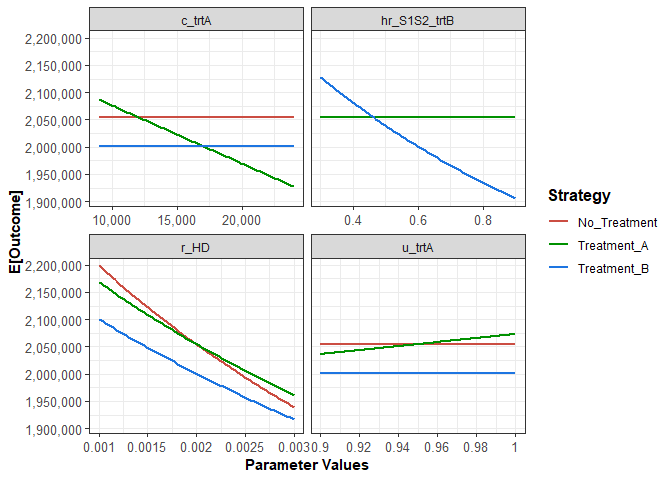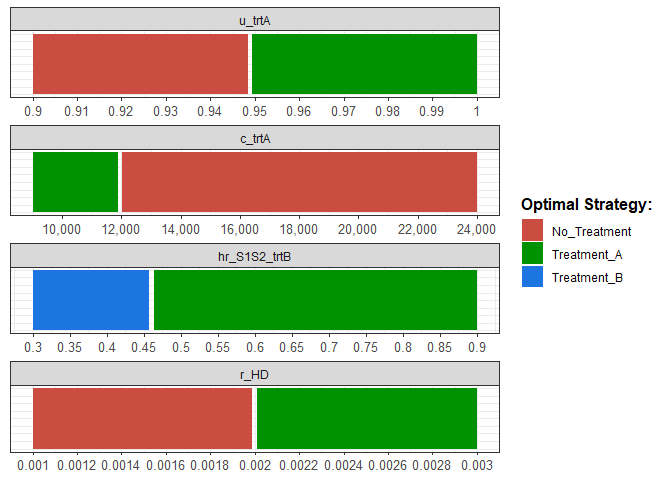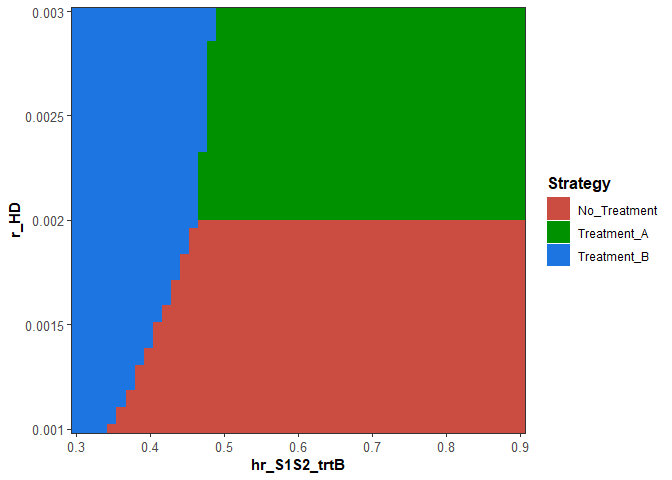# Overview

dampack has the functionality to interface with a user-defined decision model in R to conduct a deterministic sensitivity analysis (DSA) on parameters of interest. DSA is a method for assessing how the results of a decision analytic model change as a parameter of interest in varied over a specified range of values. This includes both how model outcomes change (e.g. the expected cost of each strategy) as well as how the decision changes (e.g. which strategy is the most cost-effective) with the parameter of interest.

In a DSA, the model is run for each value of the parameter of interest over the specified range, while holding all other parameter values fixed. If one parameter is varied, this is called a one-way DSA. If two parameters are varied, it is called a two-way DSA. We typically do not vary more than two parameters at a time, as the output becomes difficult to visualize and interpret. A more comprehensive assessment of how model outptus depend on model parameters can be done through a probabilistic sensitivity analysis (PSA) (type vignette("psa_generation", package = "dampack") in the console after installing the dampack package to see a vignette describing how to use dampack for PSA).

## Decision Model Format

dampack includes functionality to generate DSA results for any user-defined decision analytic model that is written in R. The user-defined model must be written as a function takes in a list of all model parameter values as its first argument and outputs a data frame of modeled outcomes (e.g. costs, QALYs, etc.) for each strategy being evaluated by the model. The first column of the output data frame must be the strategy names (as strings) with any number of additional outcomes (costs, QALYs, infections averted, etc.) stored in subsequent columns. Thus, each row of the output data frame consists of the strategy’s name followed by the corresponding outcome values for that strategy. The user-defined model function may have additional required or optional input arguments; values for these additional arguments will need to be passed to the function through the dampack DSA functions.

When using a decision analytic model to compare different potential strategies, it is often helpful to construct two functions: the model function that reflects the dynamic process being modeled and a wrapper function that runs the model with parameters that are modified to reflect the impact of different strategies. The example below will illustrate this kind of set up.

In this example, we consider a disease that can be represented as a four-state cohort state transition model (also known as a Markov model) with the health states “Healthy”, “Sick”, “Sicker”, and “Dead”. We aptly call this model the Sick-Sicker model. Individuals who are initially healthy are at risk of becomnig sick (transition to the “Sick” state). In the “Sick” state, individuals can either recover back to “Healthy” or progress to the worse “Sicker” health state, which has a lower utility and higher costs than the “Sick” and “Healthy” states. Once in the “Sicker” health states, individuals cannot recover. In every health state, individuals have a probability of dying, which is elevated for individuals in the “Sick” and “Sicker” states relative to the “Healthy” state. Individuals who die transition to the “Dead” state. For a deeper discusison of state transition models and more complex variations of this state transition model, see Alarid-Escudero F, Krijkamp EM, Enns EA, Yang A, Hunink MGM, Pechlivanoglou P, Jalal H. Cohort state-transition models in R: A Tutorial. arXiv:200107824v1. 2020:1-31.

The Sick-Sicker model is implemented as the function run_sick_sicker_model below:

run_sick_sicker_model <- function(l_params, verbose = FALSE) {
with(as.list(l_params), {
# l_params must include:
# -- disease progression parameters (annual): r_HD, p_S1S2, hr_S1D, hr_S2D,
# -- initial cohort distribution: v_s_init
# -- vector of annual state utilities: v_state_utility = c(u_H, u_S1, u_S2, u_D)
# -- vector of annual state costs: v_state_cost = c(c_H, c_S1, c_S2, c_D)
# -- time horizon (in annual cycles): n_cyles
# -- annual discount rate: r_disc

####### SET INTERNAL PARAMETERS #########################################

# state names
v_names_states <- c("H", "S1", "S2", "D")
n_states <- length(v_names_states)

# vector of discount weights
v_dw  <- 1 / ((1 + r_disc) ^ (0:n_cycles))

# state rewards
v_state_cost <- c("H" = c_H, "S1" = c_S1, "S2" = c_S2, "D" = c_D)
v_state_utility <- c("H" = u_H, "S1" = u_S1, "S2" = u_S2, "D" = u_D)

# transition probability values
r_S1D <- hr_S1D * r_HD   # rate of death in sick state
r_S2D <- hr_S2D * r_HD   # rate of death in sicker state
p_S1D <- 1 - exp(-r_S1D) # probability of dying when sick
p_S2D <- 1 - exp(-r_S2D) # probability of dying when sicker
p_HD  <- 1 - exp(-r_HD)   # probability of dying when healthy

## Initialize transition probability matrix
# all transitions to a non-death state are assumed to be conditional on survival
m_P <- matrix(0,
nrow = n_states, ncol = n_states,
dimnames = list(v_names_states, v_names_states)) # define row and column names
## Fill in matrix
# From H
m_P["H", "H"]   <- (1 - p_HD) * (1 - p_HS1)
m_P["H", "S1"]  <- (1 - p_HD) * p_HS1
m_P["H", "D"]   <- p_HD
# From S1
m_P["S1", "H"]  <- (1 - p_S1D) * p_S1H
m_P["S1", "S1"] <- (1 - p_S1D) * (1 - (p_S1H + p_S1S2))
m_P["S1", "S2"] <- (1 - p_S1D) * p_S1S2
m_P["S1", "D"]  <- p_S1D
# From S2
m_P["S2", "S2"] <- 1 - p_S2D
m_P["S2", "D"]  <- p_S2D
# From D
m_P["D", "D"]   <- 1

# check that all transition matrix entries are between 0 and 1
if(!all(m_P <= 1 & m_P >= 0)){
stop("This is not a valid transition matrix (entries are not between 0 and 1")
} else
# check transition matrix rows add up to 1
if (!all.equal(as.numeric(rowSums(m_P)),rep(1,n_states))){
stop("This is not a valid transition matrix (rows do not sum to 1)")
}

####### INITIALIZATION #########################################
# create the cohort trace
m_Trace <- matrix(NA, nrow = n_cycles + 1 ,
ncol = n_states,
dimnames = list(0:n_cycles, v_names_states)) # create Markov trace

# create vectors of costs and QALYs
v_C <- v_Q <- numeric(length = n_cycles + 1)

############# PROCESS ###########################################

m_Trace[1, ] <- v_s_init # initialize Markov trace
v_C <- 0 # no upfront costs
v_Q <- 0 # no upfront QALYs

for (t in 1:n_cycles){ # throughout the number of cycles
m_Trace[t + 1, ] <- m_Trace[t, ] %*% m_P # calculate trace for cycle (t + 1) based on cycle t

v_C[t + 1] <- m_Trace[t + 1, ] %*% v_state_cost

v_Q[t + 1] <- m_Trace[t + 1, ] %*% v_state_utility

}

#############  PRIMARY ECONOMIC OUTPUTS  #########################

# Total discounted costs
n_tot_cost <- t(v_C) %*% v_dw

# Total discounted QALYs
n_tot_qaly <- t(v_Q) %*% v_dw

#############  OTHER OUTPUTS   ###################################

# Total discounted life-years (sometimes used instead of QALYs)
n_tot_ly <- t(m_Trace %*% c(1, 1, 1, 0)) %*% v_dw

####### RETURN OUTPUT  ###########################################
out <- list(m_Trace = m_Trace,
m_P = m_P,
l_params,
n_tot_cost = n_tot_cost,
n_tot_qaly = n_tot_qaly,
n_tot_ly = n_tot_ly)

return(out)
}
)
}

Note that this function outputs costs, QALYs, and LYs for a given set of inputs (passed as the list l_params). Different strategies can be modeled by calling run_sick_sicker_model with different parameter values reflect the impacts of those strategies. In this example, we will use the Sick-Sicker model to evaluate three strateges: “No_Treatment”, “Treatment_A”, and “Treatment_B”. The “No_Treatment” strategy is just the Sick-Sicker natural history, while both “Treatment_A” and “Treatment_B” improve some aspect of the disease. These treatments are taken whenever someone is ill (Sick or Sicker), which increases the cost of being in those states by the cost of treatment.“Treatment_A” improves the utility for those in the Sick states (but not in the Sicker state), while “Treatment_B” reduces the rate of progressing from the Sick state to the Sicker state. Note that we assume that treatment cannot be targeted to just the Sick state because we assume that for this disease it is difficult to differentiate between patients in the Sick and Sicker states. Thus treatment costs are incurred by those in the Sicker state even if they do not benefit from it.

We define a second function, simulate_strategies that calls the Sick-Sicker model with the approrpiate inputs values for these three strategies. The simulate_strategies function also takes a list of parameter values (l_params), which must include both parameters for the markov model and any parameters governing the three strategies to be evaluated. simulate_strategies outputs a data frame with costs, QALYs, life-years (LYs), and net monetary benefit (NMB) for each of the three strategies. It is this function that we will pass to dampack’s internal functions for conducting DSA.

simulate_strategies <- function(l_params, wtp = 100000){
# l_params_all must include:
# -- *** Model parameters ***
# -- disease progression parameters (annual): r_HD, p_S1S2, hr_S1D, hr_S2D,
# -- initial cohort distribution: v_s_init
# -- vector of annual state utilities: v_state_utility = c(u_H, u_S1, u_S2, u_D)
# -- vector of annual state costs: v_state_cost = c(c_H, c_S1, c_S2, c_D)
# -- time horizon (in annual cycles): n_cyles
# -- annual discount rate: r_disc
# -- *** Strategy specific parameters ***
# -- treartment costs (applied to Sick and Sicker states): c_trtA, c_trtB
# -- utility with Treatment_A (for Sick state only): u_trtA
# -- hazard ratio of progression with Treatment_B: hr_S1S1_trtB

with(as.list(l_params), {

####### SET INTERNAL PARAMETERS #########################################
# Strategy names
v_names_strat <- c("No_Treatment", "Treatment_A", "Treatment_B")
# Number of strategies
n_strat <- length(v_names_strat)

## Treatment_A
# utility impacts
u_S1_trtA <- u_trtA
# include treatment costs
c_S1_trtA <- c_S1 + c_trtA
c_S2_trtA <- c_S2 + c_trtA

## Treatment_B
# progression impacts
r_S1S2_trtB <- -log(1 - p_S1S2) * hr_S1S2_trtB
p_S1S2_trtB <- 1 - exp(-r_S1S2_trtB)
# include treatment costs
c_S1_trtB <- c_S1 + c_trtB
c_S2_trtB <- c_S2 + c_trtB

####### INITIALIZATION #########################################
# Create cost-effectiveness results data frame
df_ce <- data.frame(Strategy = v_names_strat,
Cost = numeric(n_strat),
QALY = numeric(n_strat),
LY = numeric(n_strat),
stringsAsFactors = FALSE)

######### PROCESS ##############################################
for (i in 1:n_strat){
l_params_markov <- list(n_cycles = n_cycles, r_disc = r_disc, v_s_init = v_s_init,
c_H =  c_H, c_S1 = c_S2, c_S2 = c_S1, c_D = c_D,
u_H =  u_H, u_S1 = u_S2, u_S2 = u_S1, u_D = u_D,
r_HD = r_HD, hr_S1D = hr_S1D, hr_S2D = hr_S2D,
p_HS1 = p_HS1, p_S1H = p_S1H, p_S1S2 = p_S1S2)

if (v_names_strat[i] == "Treatment_A"){
l_params_markov$u_S1 <- u_S1_trtA l_params_markov$c_S1 <- c_S1_trtA
l_params_markov$c_S2 <- c_S2_trtA } else if(v_names_strat[i] == "Treatment_B"){ l_params_markov$p_S1S2 <- p_S1S2_trtB
l_params_markov$c_S1 <- c_S1_trtB l_params_markov$c_S2   <- c_S2_trtB
}

l_result <- run_sick_sicker_model(l_params_markov)

df_ce[i, c("Cost", "QALY", "LY")] <- c(l_result$n_tot_cost, l_result$n_tot_qaly,
l_result$n_tot_ly) df_ce[i, "NMB"] <- l_result$n_tot_qaly * wtp - l_result$n_tot_cost } return(df_ce) }) } To demonstrate the simulate_strategies function, we define a list of model and strategy parameters. We call this our basecase list of parameters, as these will be the default parameter values used when conducting the DSA. my_params_basecase <- list(p_HS1 = 0.15, p_S1H = 0.5, p_S1S2 = 0.105, r_HD = 0.002, hr_S1D = 3, hr_S2D = 10, hr_S1S2_trtB = 0.6, c_H = 2000, c_S1 = 4000, c_S2 = 15000, c_D = 0, c_trtA = 12000, c_trtB = 13000, u_H = 1, u_S1 = 0.75, u_S2 = 0.5, u_D = 0, u_trtA = 0.95, n_cycles = 75, v_s_init = c(1, 0, 0, 0), r_disc = 0.03) If we then call simulate_strategies with the list my_params_basecase, we will get a data frame of strategy outcomes: df_ce <- simulate_strategies(my_params_basecase) df_ce #> Strategy Cost QALY LY NMB #> 1 No_Treatment 112005.4 21.66567 26.24113 2054562 #> 2 Treatment_A 276350.4 23.31164 26.24113 2054814 #> 3 Treatment_B 248990.2 22.49849 26.95238 2000859 ## Generating DSA results Next, we will use the functionality within dampack to generate one- and two-way DSAs using our user-defined function, simulate_strategies. A simple one-way DSA starts with choosing a model parameter to be investigated. Next, the modeler specifies a range for this parameter and the number of evenly spaced points along this range at which to evaluate model outcomes. The model is then run for each element of the vector of parameter values by setting the parameter of interest to the value, holding all other model parameters at their default base case values. The dampack functions run_owsa_det and run_twsa_det are used to execute one- and two-way DSA, respectively. ## One-way DSA generation To execute a one-way DSA, we first create a data frame that contains the list of parameter names to be varied (first column) and the minimum (second column) and maximum (third column) values of the range of values for each parameter. In the example below, we will conduct four one-way DSAs separately on four different parameters: the utility benefit of Treatment_A; the cost of Treatment_A; the reduction, as a hazard ratio, on the rate of progressing from Sick to Sicker with Treatment_B; and the rate of dying from the Healthy state. my_owsa_params_range <- data.frame(pars = c("u_trtA", "c_trtA", "hr_S1S2_trtB", "r_HD"), min = c(0.9, 9000, 0.3, 0.001), max = c(1, 24000, 0.9, 0.003)) To conduct the one-way DSA, we then call the function run_owsa_det with my_owsa_params_range, specifying the parameters to be varied and over which ranges, and my_params_basecase as the fixed parameter values to be used in the model when a parameter is not being explicitly varied in the one-way sensitivity analysis. Note that the parameters specified in my_owsa_params_range$pars are a subset of the parameters listed in my_params_basecase.

Additional inputs are the number of equally-spaced samples (nsamp) to be used between the specified minimum and maximum of each range, the user-defined function (FUN) to be called to generate model outcomes for each strategy, the vector of outcomes to be stored (must be outcomes generated by the data frame output of the function passed in FUN), and the vector of strategy names to be evaluated.

The input progress = TRUE allows the user to see a progress bar as the DSA is conducted. When many parameters are being varied, nsamp is large, and/or the user-defined function is computationally burdensome, the DSA may take a noticeable amount of time to compute and the progress display is recommended.

library(dampack)
l_owsa_det <- run_owsa_det(params_range = my_owsa_params_range,
params_basecase = my_params_basecase,
nsamp = 100,
FUN = simulate_strategies,
outcomes = c("Cost", "QALY", "LY", "NMB"),
strategies = c("No_Treatment", "Treatment_A", "Treatment_B"),
progress = FALSE)

Because we have defined multiple parameters in my_owsa_params_range, we have instructed run_owsa_det to execute a series of separate one-way sensitivity analyses and compile the results into a single owsa object for each requested outcome. When only one outcome is specified run_owsa_det returns a owsa data frame. When more than one outcome is specified, run_owsa_det returns a list containing one owsa data frame for each outcome. To access the owsa object corresponding to a given outcome, one can select the list item with the name “owsa_”. For example, the owsa object associated with the NMB outcome can be accessed as l_owsa_det$owsa_NMB. Each owsa object returned by run_owsa_det is a data frame with four columns: parameter, strategy, param_val, and outcome_val. For each row, param_val is the value used for the parameter listed in parameter and outcome_val is the value of the specified outcome for the strategy listed in strategy. The owsa object can now be visualized using the functionality within dampack, which includes an owsa plot method and a visualization of the optimal strategy over each parameter range (function owsa_opt_strat). # Select the net monetary benefit (NMB) owsa object my_owsa_NMB <- l_owsa_det$owsa_NMB

# Plot outcome of each strategy over each parameter range
plot(my_owsa_NMB,
n_x_ticks = 3)# Visualize optimal strategy (max NMB) over each parameter range
owsa_opt_strat(my_owsa_NMB)## Two-way DSA Generation

A two-way DSA is used to assess how model outcomes vary over specified ranges of two model parameters jointly. Similar to a one-way DSA, we first create a data frame with the (two) parameters that we wish to vary and their individual ranges. This data frame must have exactly two rows since the two-way DSA requires exactly two parameters.

my_twsa_params_range <- data.frame(pars = c("hr_S1S2_trtB", "r_HD"),
min = c(0.3, 0.001),
max = c(0.9, 0.003))

To conduct the two-way DSA, we then call the function run_twsa_det with my_twsa_params_range. The general format of the function arguments for run_twsa_det are the same as those for run_owsa_det. In run_twsa_det, equally spaced sequences of length nsamp are created for the two parameters based on the inputs provided in the params_range argument. These two sequences of parameter values define an nsamp by nsamp grid over which FUN is applied to produce outcomes for every combination of the two parameters.

l_twsa_det <- run_twsa_det(params_range = my_twsa_params_range,
params_basecase = my_params_basecase,
nsamp = 50,
FUN = simulate_strategies,
outcomes = c("Cost", "QALY", "NMB"),
strategies = c("No_Treatment", "Treatment_A", "Treatment_B"),
progress = FALSE)

Like in the one-way DSA, if only one outcome is specified, then run_twsa_det will return a twsa data frame. If more than one outcome is specified, run_twsa_det returns a list containing one twsa object for each outcome. To access the twsa object corresponding to a given outcome, one can select the list item with the name “twsa_”. For example, the twsa object associated with the NMB outcome can be accessed as l_twsa_det$owsa_NMB. Each twsa object returned by run_twsa_det is a data frame with four columns. The first two columns are named according to the two parameters specified in params_range. Each row is the value of that parameter corresponding to the outcome value in the outcome_val (fourth) column for the strategy listed in the strategy (third) column. The twsa object can now be visualized using the functionality within dampack. The twsa plot method shows the optimal strategy as a function of the two parameters being varied in the two-way DSA, which is the primary way that two-way analyses are reported. my_twsa_NMB <- l_twsa_det$twsa_NMB

# plot optimal strategy (max NMB) as a function of the two parameters varied in the two-way DSA
plot(my_twsa_NMB)